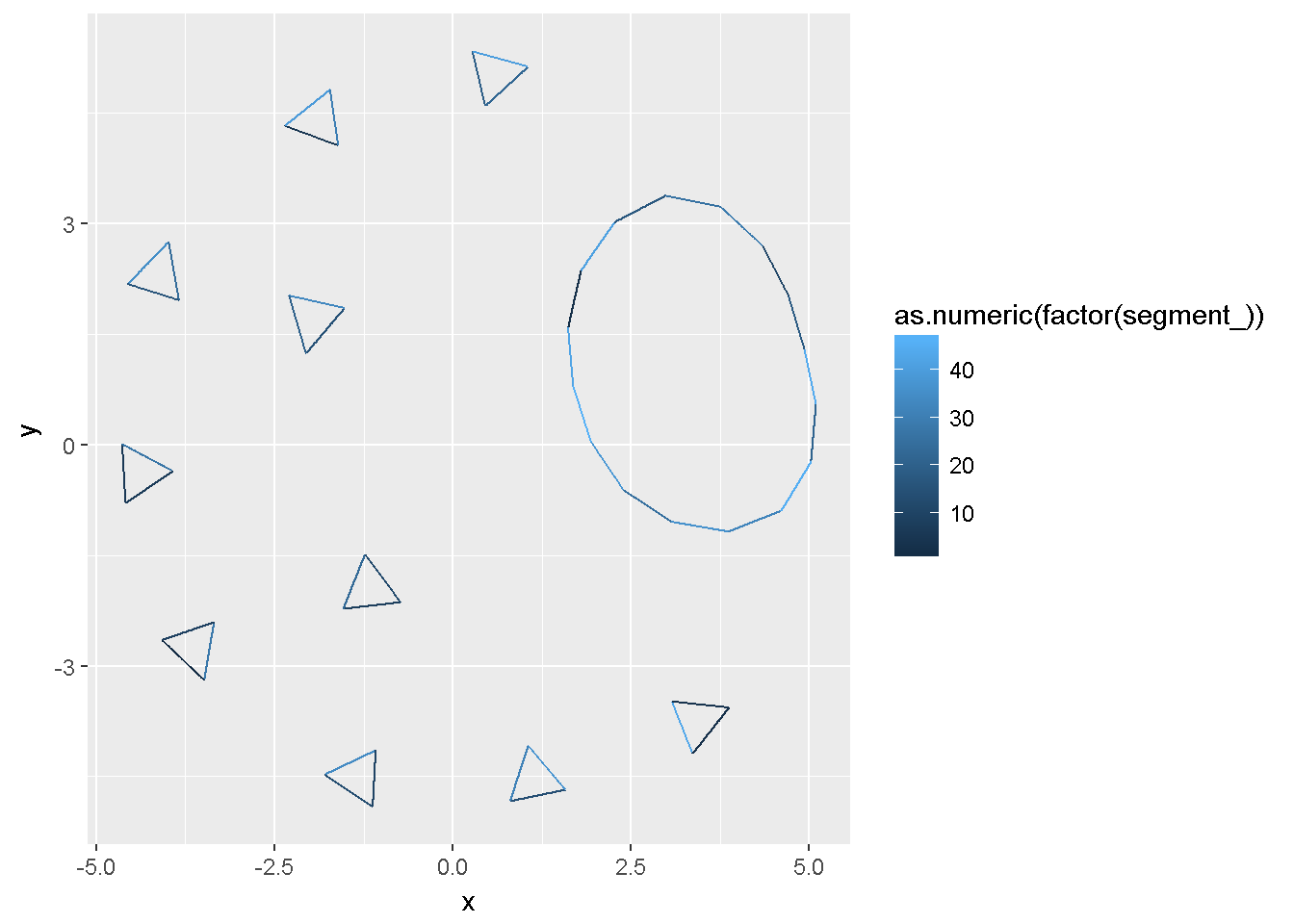library(ggraph)
## Loading required package: ggplot2
library(igraph)
##
## Attaching package: 'igraph'
## The following objects are masked from 'package:stats':
##
##     decompose, spectrum
## The following object is masked from 'package:base':
##
##     union
#devtools::install_github(c("mdsumner/sc", "mdsumner/scsf"))
library(scsf)
## Loading required package: sc
data("minimal_mesh")
prim <- sc::PRIMITIVE(minimal_mesh)
library(dplyr)
##
## Attaching package: 'dplyr'
## The following objects are masked from 'package:igraph':
##
##     %>%, as_data_frame, groups, union
## The following objects are masked from 'package:stats':
##
##     filter, lag
## The following objects are masked from 'package:base':
##
##     intersect, setdiff, setequal, union
mm_g <- graph_from_data_frame(prim$segment %>% rename(from = .vertex0, to = .vertex1)) library(sf) ## Linking to GEOS 3.5.0, GDAL 2.1.1, proj.4 4.9.3 nc <- st_read(system.file("shape/nc.shp", package="sf"), quiet = TRUE) plot(nc[4:10, 1:2])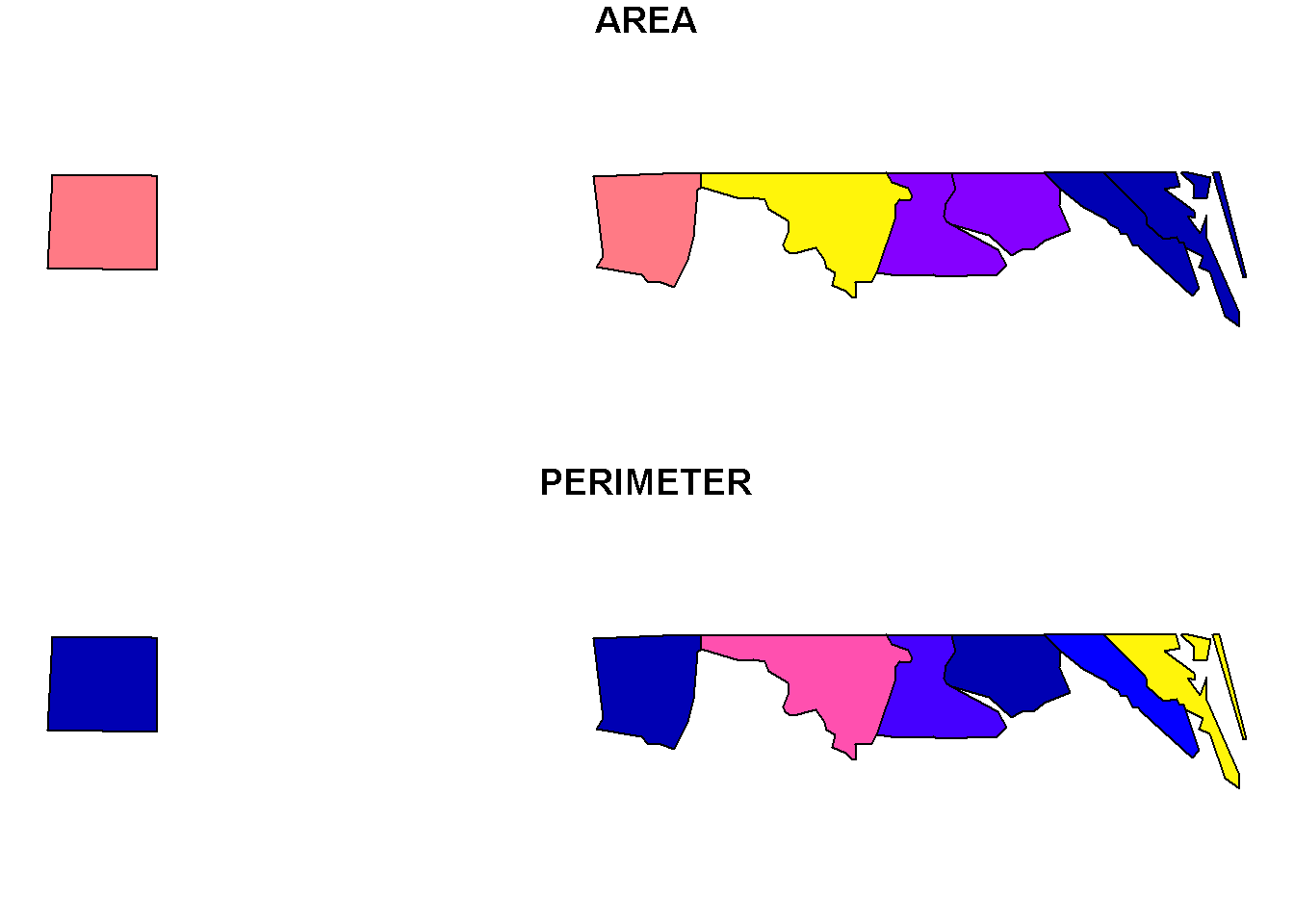prim <- PRIMITIVE(nc[4:10, ]) nc_g <- graph_from_data_frame(prim$segment %>% rename(from = .vertex0, to = .vertex1))

ggraph(nc_g, layout = 'kk') +
geom_edge_link(aes(colour = as.numeric(factor(segment_))))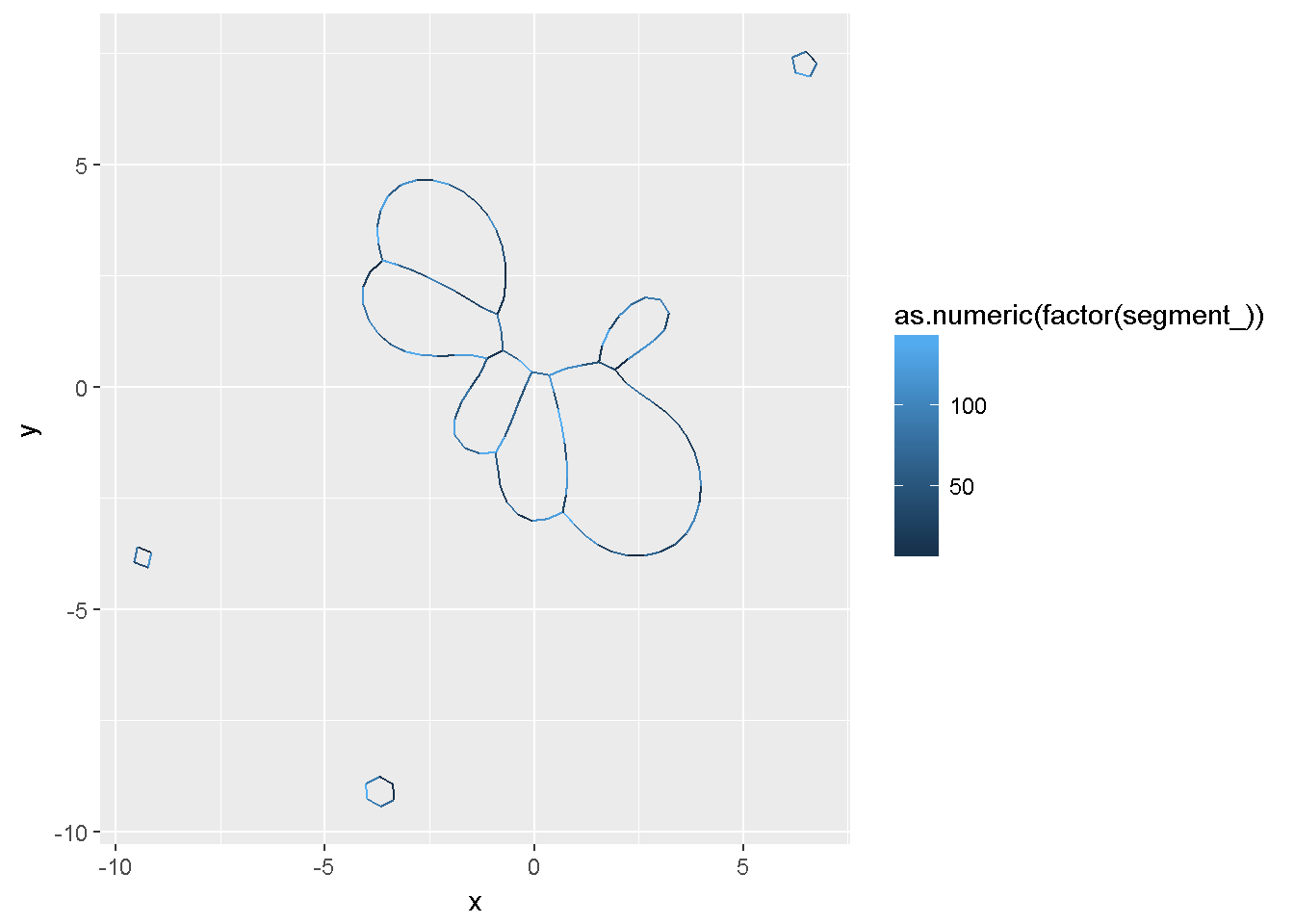ggraph(nc_g, layout = 'kk') +
geom_edge_link(aes(colour = path_))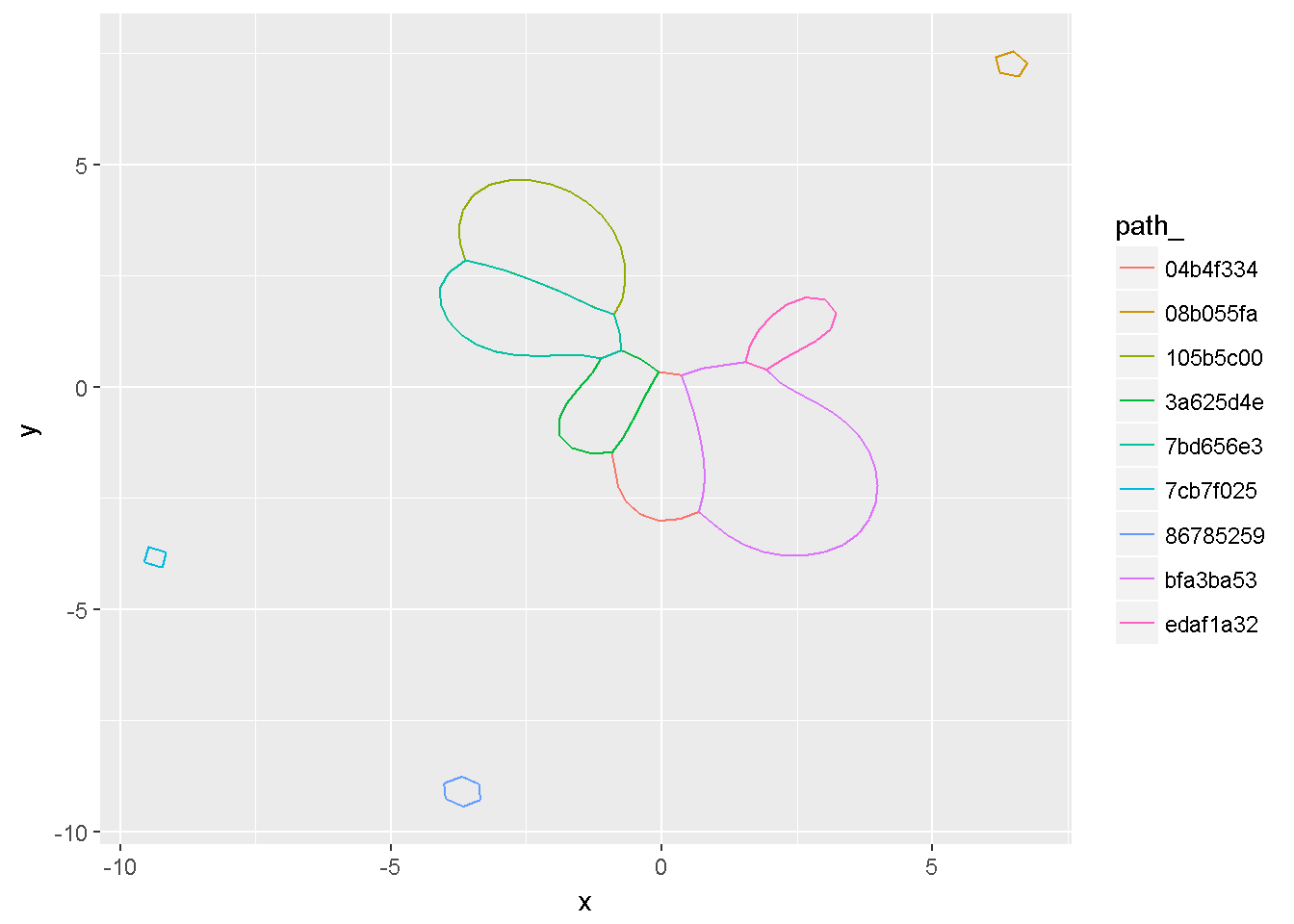##devtools::install_github("mdsumner/sfdct")
#data("antarctica", package = "sfdct")

data("wrld_simpl", package = "maptools")
oz <- sp::disaggregate(subset(wrld_simpl, NAME == "Australia"))
tas <- st_as_sf(subset(oz, sp::coordinates(oz)[, 2] < -40))
plot(st_geometry(tas))prim <- PRIMITIVE(tas)
tas_g <- graph_from_data_frame(prim\$segment %>% rename(from = .vertex0, to = .vertex1))
ggraph(tas_g, layout = 'kk') +
geom_edge_link(aes(colour = path_))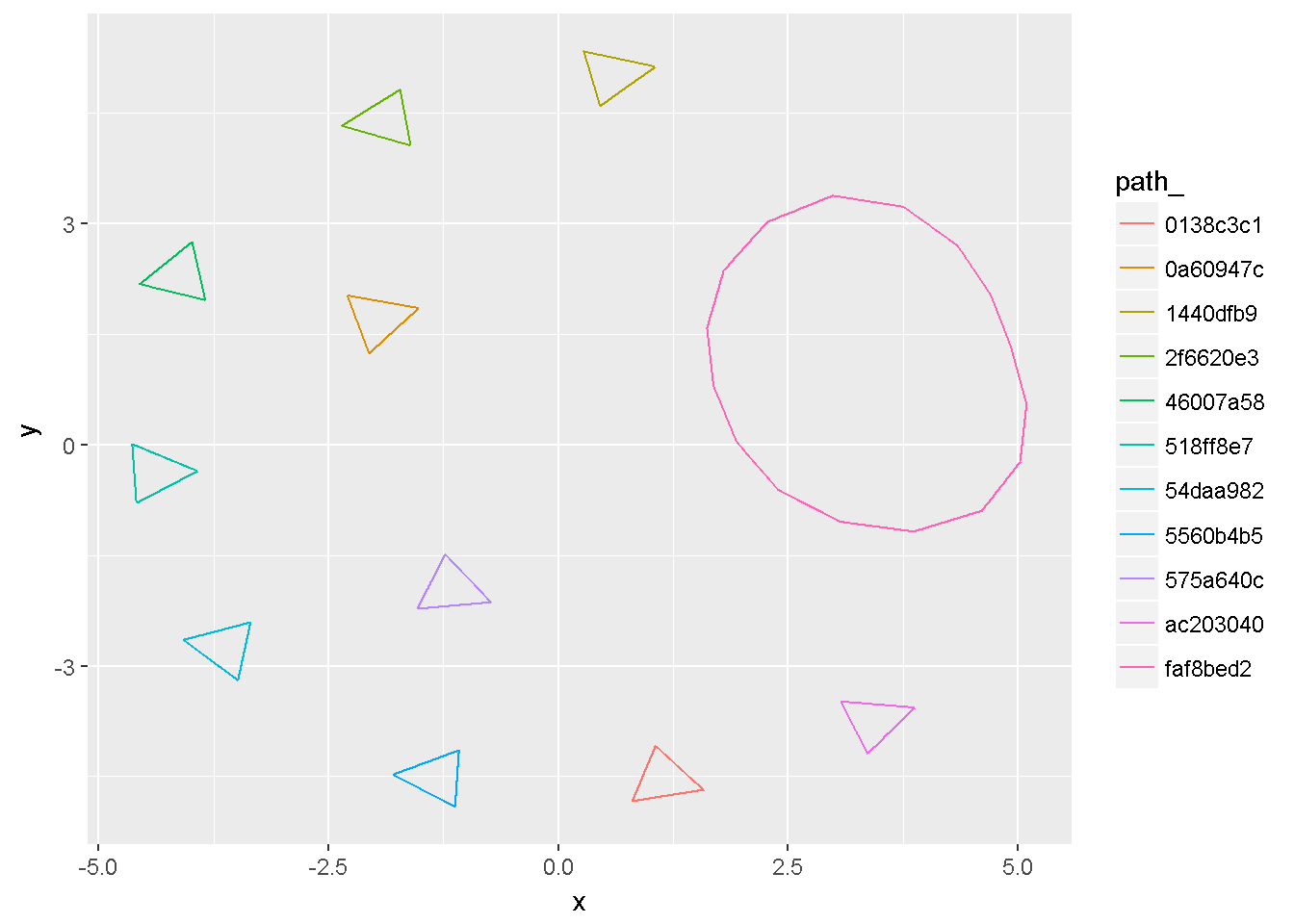ggraph(tas_g, layout = 'kk') +
geom_edge_link(aes(colour = as.numeric(factor(segment_))))